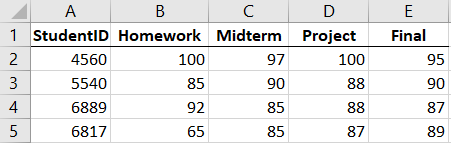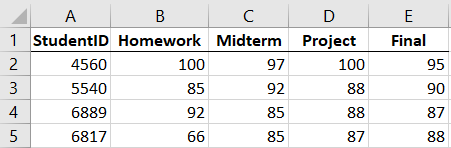## Introduction to Comparing Pandas DataFrames in Python

A common occurrence in data science is the validation of data between two executions of code. For example, suppose a data scientist performed an analysis to produce a data set, and it is your job to replicate that data set to validate the method. Given two Pandas DataFrames, how do we verify that they contain the same data? We can perform a simple True/False check on the two DataFrames to determine their structural equality using the Pandas `equal` method, and if some differences exist between them, we can create a new data frame for comparing the individual differences. The ability to compare DataFrames and mark the differences is often overlooked in Pandas tutorials, but it truly is the most valuable part of any data comparison.

For this tutorial we’ll be comparing the following two DataFrames containing slightly different values of student gradesSuppose we now want to compare these spreadsheets to determine if there are differences, and to find out what those differences are. First we’ll need to import the Excel data into two Pandas DataFrames:

``````import pandas as pd

Now that our data is imported, we can compare these two DataFrames with a few different Python Pandas operations.

## Simple DataFrame Comparison: equals Method

If we want to perform a simple comparison between dataframes to determine whether or not they’re identical, we should use the equals DataFrame method. This method has the format:

``[pandas.DataFrame].equals([pandas.DataFrame])``

where `[pandas.DataFrame]` is a particular Pandas DataFrame you wish to compare to another. The `equals` method returns a boolean of True if the DataFrames are identical or False if the DataFrames differ.

``````grades1.equals(grades1)  # Compare a DataFrame to itself
> True
> False``````

I put together a Python Developer Kit with over 100 pre-built Python scripts covering data structures, Pandas, NumPy, Seaborn, machine learning, file processing, web scraping and a whole lot more - and I want you to have it for free. Enter your email address below and I'll send a copy your way.

Yes, I'll take a free Python Developer Kit

## Identifying DataFrame Differences

Once we’ve determined that the DataFrames are not identical using the `equals` method, we can look further to determine the exact differences between the two sets of data. Pandas does not contain a standalone “diff” method for comparison of differences, however we can easily code one ourselves using only a few lines of Python code:

``````gradeBool = (grades1 != grades2).stack()  # Create Frame of comparison booleans
>                     Old  New
> StudentID
> 5540      Midterm    90   92
> 6817      Homework   65   66
>           Final      89   88``````

Let’s break down how the above code successfully compares our two DataFrames for differences.

1. The grades1 and grades2 DataFrames are the two DataFrames we want to compare in our Python Pandas code.
2. The gradeBool DataFrame we created contains Boolean values indicating if the individual elements between the two frames are the same.1 The `stack` method will then convert the DataFrame into a “long” form, where each index-column pair is represented as its own row.
3. The gradediff DataFrame we created is a horizontal concatenation of the two original data frames, selecting only the differences identified by the gradeBool DataFrame. The `concat` function with the option `axis=1` will indicate that the resulting DataFrame should be the two given DataFrames, joined by index with separate columns. Again, the `stack` method is used to convert each DataFrame into a “long” format to ease identification of the differences
4. The resulting gradediff DataFrame has no column names to indicate which column contains which set of data, so we set the names directly to “Old” and “New”. Feel free to change the names to something like “Grades1” and “Grades2”.

The resulting difference DataFrame we created makes it easy to identify which index and column between the two DataFrames are different, and it shows us the corresponding value changes.

We can further speed up the process by including all of the above code into a Python function, which we can use to easily calculate DataFrame differences anytime we need to:

``````import pandas as pd  # Make sure import aliases are consistent
def dfDiff(oldFrame, newFrame):
dfBool = (oldFrame != newFrame).stack()
diff = pd.concat([oldFrame.stack()[dfBool],
newFrame.stack()[dfBool]], axis=1)
diff.columns=["Old", "New"]
return diff
>                     Old  New
> StudentID
> 5540      Midterm    90   92
> 6817      Homework   65   66
>           Final      89   88``````